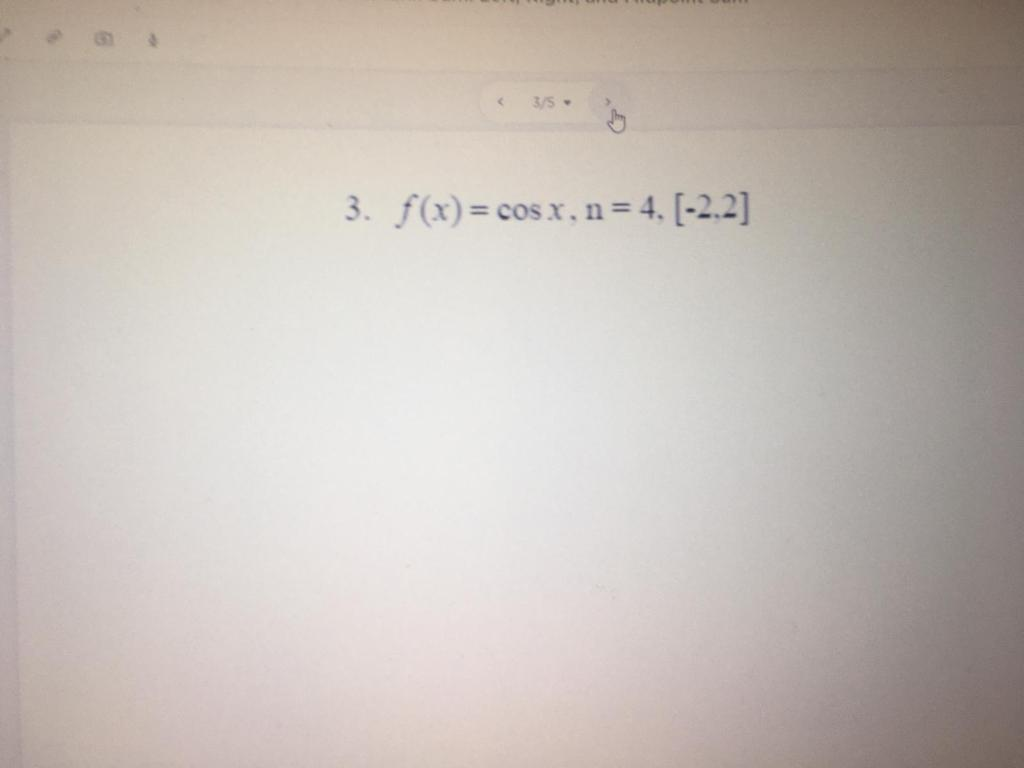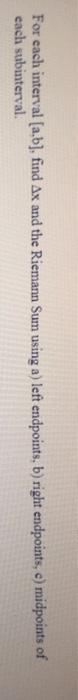Create an Account

Home / Questions / 35 3. f(x) = cos x, n = 4, 1-2.2] For each interval [a,b], find Ax and the Riemann Sum usi...

35 3. f(x) = cos x, n = 4, 1-2.2] For each interval [a,b], find Ax and the Riemann Sum using a) left endpoints, b) right endpoints, e) midpoints of each subinterval.

35 3. f(x) = cos x, n = 4, 1-2.2]
For each interval [a,b], find Ax and the Riemann Sum using a) left endpoints, b) right endpoints, e) midpoints of each subinterval.May 06 2021 View more View LessSubscribe To Get Solution